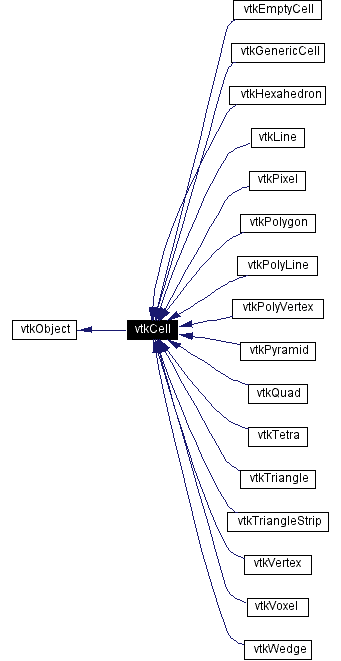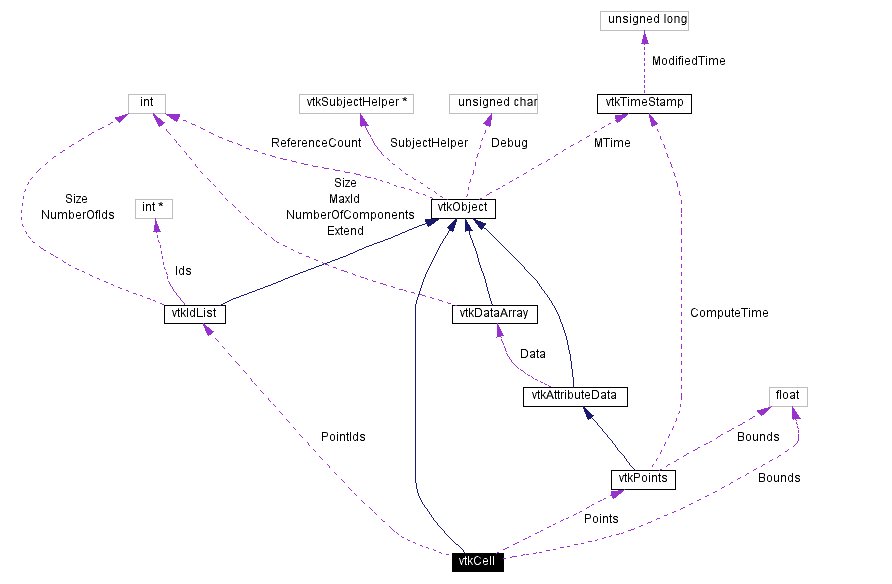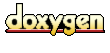Main Page   Class Hierarchy   Alphabetical List   Compound List   File List   Compound Members   File Members   Related Pages

# vtkCell Class Reference

abstract class to specify cell behavior. More...

`#include <vtkCell.h>`

Inheritance diagram for vtkCell:[legend]
Collaboration diagram for vtkCell:[legend]
List of all members.

## Public Methods

virtual const char * GetClassName ()
virtual int IsA (const char *type)
void PrintSelf (ostream &os, vtkIndent indent)
void Initialize (int npts, int *pts, vtkPoints *p)
virtual vtkCell * MakeObject ()=0
virtual void ShallowCopy (vtkCell *c)
virtual void DeepCopy (vtkCell *c)
virtual int GetCellType ()=0
virtual int GetCellDimension ()=0
virtual int GetInterpolationOrder ()
vtkPointsGetPoints ()
int GetNumberOfPoints ()
virtual int GetNumberOfEdges ()=0
virtual int GetNumberOfFaces ()=0
vtkIdListGetPointIds ()
int GetPointId (int ptId)
virtual vtkCell * GetEdge (int edgeId)=0
virtual vtkCell * GetFace (int faceId)=0
virtual int CellBoundary (int subId, float pcoords, vtkIdList *pts)=0
virtual int EvaluatePosition (float x, float *closestPoint, int &subId, float pcoords, float &dist2, float *weights)=0
virtual void EvaluateLocation (int &subId, float pcoords, float x, float *weights)=0
virtual void Contour (float value, vtkScalars *cellScalars, vtkPointLocator *locator, vtkCellArray *verts, vtkCellArray *lines, vtkCellArray *polys, vtkPointData *inPd, vtkPointData *outPd, vtkCellData *inCd, int cellId, vtkCellData *outCd)=0
virtual void Clip (float value, vtkScalars *cellScalars, vtkPointLocator *locator, vtkCellArray *connectivity, vtkPointData *inPd, vtkPointData *outPd, vtkCellData *inCd, int cellId, vtkCellData *outCd, int insideOut)=0
virtual int IntersectWithLine (float p1, float p2, float tol, float &t, float x, float pcoords, int &subId)=0
virtual int Triangulate (int index, vtkIdList *ptIds, vtkPoints *pts)=0
virtual void Derivatives (int subId, float pcoords, float *values, int dim, float *derivs)=0
void GetBounds (float bounds)
float * GetBounds ()
float GetLength2 ()
virtual int GetParametricCenter (float pcoords)
void DeepCopy (vtkCell &c)
void ShallowCopy (vtkCell &c)

## Static Public Methods

int IsTypeOf (const char *type)
vtkCell * SafeDownCast (vtkObject *o)
char HitBBox (float bounds, float origin, float dir, float coord, float &t)

## Public Attributes

vtkPointsPoints
vtkIdListPointIds

## Protected Methods

vtkCell ()
~vtkCell ()
vtkCell (const vtkCell &)
void operator= (const vtkCell &)

float Bounds 

## Detailed Description

abstract class to specify cell behavior.

Date:
2000/12/10 20:08:06
Revision:
1.64

vtkCell is an abstract class that specifies the interfaces for data cells. Data cells are simple topological elements like points, lines, polygons, and tetrahedra of which visualization datasets are composed. In some cases visualization datasets may explicitly represent cells (e.g., vtkPolyData, vtkUnstructuredGrid), and in some cases, the datasets are implicitly composed of cells (e.g., vtkStructuredPoints).

Warning:
The define VTK_CELL_SIZE is a parameter used to construct cells and provide a general guideline for controlling object execution. This parameter is not a hard boundary: you can create cells with more points.
vtkHexahedron vtkLine vtkPixel vtkPolyLine vtkPolyVertex vtkPolygon vtkQuad vtkTetra vtkTriangle vtkTriangleStrip vtkVertex vtkVoxel vtkWedge vtkPyramid
Examples:
vtkCell (examples)

Definition at line 85 of file vtkCell.h.

## Constructor & Destructor Documentation

 vtkCell::vtkCell ( ) ` [protected]`

 vtkCell::~vtkCell ( ) ` [protected]`

 vtkCell::vtkCell ( const vtkCell & ) ` [inline, protected]`
 Definition at line 285 of file vtkCell.h.

## Member Function Documentation

 virtual const char* vtkCell::GetClassName ( ) ` [virtual]`
 Return the class name as a string. This method is defined in all subclasses of vtkObject with the vtkTypeMacro found in vtkSetGet.h. Reimplemented from vtkObject.

 int vtkCell::IsTypeOf ( const char * type ) ` [static]`
 Return 1 if this class type is the same type of (or a subclass of) the named class. Returns 0 otherwise. This method works in combination with vtkTypeMacro found in vtkSetGet.h. Reimplemented from vtkObject.

 virtual int vtkCell::IsA ( const char * type ) ` [virtual]`
 Return 1 if this class is the same type of (or a subclass of) the named class. Returns 0 otherwise. This method works in combination with vtkTypeMacro found in vtkSetGet.h. Reimplemented from vtkObject.

 vtkCell* vtkCell::SafeDownCast ( vtkObject * o ) ` [static]`
 Will cast the supplied object to vtkObject* is this is a safe operation (i.e., a safe downcast); otherwise NULL is returned. This method is defined in all subclasses of vtkObject with the vtkTypeMacro found in vtkSetGet.h. Reimplemented from vtkObject.

 void vtkCell::PrintSelf ( ostream & os, vtkIndent indent ) ` [virtual]`
 Methods invoked by print to print information about the object including superclasses. Typically not called by the user (use Print() instead) but used in the hierarchical print process to combine the output of several classes. Reimplemented from vtkObject.

 void vtkCell::Initialize ( int npts, int * pts, vtkPoints * p )
 Initialize cell from outside with point ids and point coordinates specified.

 virtual vtkCell* vtkCell::MakeObject ( ) ` [pure virtual]`
 Create concrete copy of this cell. Initially, the copy is made by performing a ShallowCopy() operation.

 virtual void vtkCell::ShallowCopy ( vtkCell * c ) ` [virtual]`
 Copy this cell by reference counting the internal data structures. This is safe if you want a "read-only" copy. If you modify the cell you might wish to use DeepCopy(). Reimplemented in vtkGenericCell. Referenced by vtkGenericCell::ShallowCopy().

 virtual void vtkCell::DeepCopy ( vtkCell * c ) ` [virtual]`
 Copy this cell by completely copying internal data structures. This is slower but safer than ShallowCopy(). Reimplemented in vtkGenericCell. Referenced by vtkGenericCell::DeepCopy().

 virtual int vtkCell::GetCellType ( ) ` [pure virtual]`
 Return the type of cell.

 virtual int vtkCell::GetCellDimension ( ) ` [pure virtual]`
 Return the topological dimensional of the cell (0,1,2, or 3).

 virtual int vtkCell::GetInterpolationOrder ( ) ` [inline, virtual]`
 Return the interpolation order of the cell. Usually linear. Reimplemented in vtkGenericCell. Definition at line 115 of file vtkCell.h.

 vtkPoints* vtkCell::GetPoints ( ) ` [inline]`
 Get the point coordinates for the cell. Definition at line 118 of file vtkCell.h.

 int vtkCell::GetNumberOfPoints ( ) ` [inline]`
 Return the number of points in the cell. Definition at line 121 of file vtkCell.h. Referenced by vtkTriangleStrip::GetNumberOfEdges(), vtkPolygon::GetNumberOfEdges(), and vtkCellArray::InsertNextCell().

 virtual int vtkCell::GetNumberOfEdges ( ) ` [pure virtual]`
 Return the number of edges in the cell.

 virtual int vtkCell::GetNumberOfFaces ( ) ` [pure virtual]`
 Return the number of faces in the cell.

 vtkIdList* vtkCell::GetPointIds ( ) ` [inline]`
 Return the list of point ids defining the cell. Definition at line 130 of file vtkCell.h.

 int vtkCell::GetPointId ( int ptId ) ` [inline]`
 For cell point i, return the actual point id. Definition at line 133 of file vtkCell.h.

 virtual vtkCell* vtkCell::GetEdge ( int edgeId ) ` [pure virtual]`
 Return the edge cell from the edgeId of the cell.

 virtual vtkCell* vtkCell::GetFace ( int faceId ) ` [pure virtual]`
 Return the face cell from the faceId of the cell. Reimplemented in vtkEmptyCell, vtkGenericCell, vtkHexahedron, vtkLine, vtkPixel, vtkPolygon, vtkPyramid, vtkQuad, vtkTetra, vtkTriangle, vtkVertex, vtkVoxel, and vtkWedge.

 virtual int vtkCell::CellBoundary ( int subId, float pcoords, vtkIdList * pts ) ` [pure virtual]`
 Given parametric coordinates of a point, return the closest cell boundary, and whether the point is inside or outside of the cell. The cell boundary is defined by a list of points (pts) that specify a face (3D cell), edge (2D cell), or vertex (1D cell). If the return value of the method is != 0, then the point is inside the cell.

 virtual int vtkCell::EvaluatePosition ( float x, float * closestPoint, int & subId, float pcoords, float & dist2, float * weights ) ` [pure virtual]`
 Given a point x return inside(=1) or outside(=0) cell; evaluate parametric coordinates, sub-cell id (!=0 only if cell is composite), distance squared of point x to cell (in particular, the sub-cell indicated), closest point on cell to x (unless closestPoint is null, in which case, the closest point and dist2 are not found), and interpolation weights in cell. (The number of weights is equal to the number of points defining the cell). Note: on rare occasions a -1 is returned from the method. This means that numerical error has occurred and all data returned from this method should be ignored. Also, inside/outside is determine parametrically. That is, a point is inside if it satisfies parametric limits. This can cause problems for cells of topological dimension 2 or less, since a point in 3D can project onto the cell within parametric limits but be "far" from the cell. Thus the value dist2 may be checked to determine true in/out.

 virtual void vtkCell::EvaluateLocation ( int & subId, float pcoords, float x, float * weights ) ` [pure virtual]`
 Determine global coordinate (x) from subId and parametric coordinates. Also returns interpolation weights. (The number of weights is equal to the number of points in the cell.)

 virtual void vtkCell::Contour ( float value, vtkScalars * cellScalars, vtkPointLocator * locator, vtkCellArray * verts, vtkCellArray * lines, vtkCellArray * polys, vtkPointData * inPd, vtkPointData * outPd, vtkCellData * inCd, int cellId, vtkCellData * outCd ) ` [pure virtual]`
 Generate contouring primitives. The scalar list cellScalars are scalar values at each cell point. The point locator is essentially a points list that merges points as they are inserted (i.e., prevents duplicates). Contouring primitives can be vertices, lines, or polygons. It is possible to interpolate point data along the edge by providing input and output point data - if outPd is NULL, then no interpolation is performed. Also, if the output cell data is non-NULL, the cell data from the contoured cell is passed to the generated contouring primitives. (Note: the CopyAllocate() method must be invoked on both the output cell and point data. The cellId refers to the cell from which the cell data is copied.)

 virtual void vtkCell::Clip ( float value, vtkScalars * cellScalars, vtkPointLocator * locator, vtkCellArray * wedges, vtkPointData * inPd, vtkPointData * outPd, vtkCellData * inCd, int cellId, vtkCellData * outCd, int insideOut ) ` [pure virtual]`
 Cut (or clip) the cell based on the input cellScalars and the specified value. The output of the clip operation will be one or more cells of the same topological dimension as the original cell. The flag insideOut controls what part of the cell is considered inside - normally cell points whose scalar value is greater than "value" are considered inside. If insideOut is on, this is reversed. Also, if the output cell data is non-NULL, the cell data from the clipped cell is passed to the generated contouring primitives. (Note: the CopyAllocate() method must be invoked on both the output cell and point data. The cellId refers to the cell from which the cell data is copied.)

 virtual int vtkCell::IntersectWithLine ( float p1, float p2, float tol, float & t, float x, float pcoords, int & subId ) ` [pure virtual]`
 Intersect with a ray. Return parametric coordinates (both line and cell) and global intersection coordinates, given ray definition and tolerance. The method returns non-zero value if intersection occurs.

 virtual int vtkCell::Triangulate ( int index, vtkIdList * ptIds, vtkPoints * pts ) ` [pure virtual]`
 Generate simplices of proper dimension. If cell is 3D, tetrahedron are generated; if 2D triangles; if 1D lines; if 0D points. The form of the output is a sequence of points, each n+1 points (where n is topological cell dimension) defining a simplex. The index is a parameter that controls which triangulation to use (if more than one is possible). If numerical degeneracy encountered, 0 is returned, otherwise 1 is returned.

 virtual void vtkCell::Derivatives ( int subId, float pcoords, float * values, int dim, float * derivs ) ` [pure virtual]`
 Compute derivatives given cell subId and parametric coordinates. The values array is a series of data value(s) at the cell points. There is a one-to-one correspondence between cell point and data value(s). Dim is the number of data values per cell point. Derivs are derivatives in the x-y-z coordinate directions for each data value. Thus, if computing derivatives for a scalar function in a hexahedron, dim=1, 8 values are supplied, and 3 deriv values are returned (i.e., derivatives in x-y-z directions). On the other hand, if computing derivatives of velocity (vx,vy,vz) dim=3, 24 values are supplied ((vx,vy,vz)1, (vx,vy,vz)2, ....()8), and 9 deriv values are returned ((d(vx)/dx),(d(vx)/dy),(d(vx)/dz), (d(vy)/dx),(d(vy)/dy), (d(vy)/dz), (d(vz)/dx),(d(vz)/dy),(d(vz)/dz)).

 void vtkCell::GetBounds ( float bounds )
 Compute cell bounding box (xmin,xmax,ymin,ymax,zmin,zmax). Copy result into user provided array.

 float* vtkCell::GetBounds ( )
 Compute cell bounding box (xmin,xmax,ymin,ymax,zmin,zmax). Return pointer to array of six float values.

 float vtkCell::GetLength2 ( )
 Compute Length squared of cell (i.e., bounding box diagonal squared).

 virtual int vtkCell::GetParametricCenter ( float pcoords ) ` [virtual]`
 Return center of the cell in parametric coordinates. Note that the parametric center is not always located at (0.5,0.5,0.5). The return value is the subId that the center is in (if a composite cell). If you want the center in x-y-z space, invoke the EvaluateLocation() method. Reimplemented in vtkGenericCell, vtkPolyLine, vtkPolyVertex, vtkPyramid, vtkTetra, vtkTriangle, vtkTriangleStrip, and vtkWedge.

 char vtkCell::HitBBox ( float bounds, float origin, float dir, float coord, float & t ) ` [static]`
 Bounding box intersection modified from Graphics Gems Vol I. The method returns a non-zero value if the bounding box is hit. Origin starts the ray, dir is the vector components of the ray in the x-y-z directions, coord is the location of hit, and t is the parametric coordinate along line. (Notes: the intersection ray dir is NOT normalized. Valid intersections will only occur between 0<=t<=1.)

 void vtkCell::DeepCopy ( vtkCell & c ) ` [inline]`
 For legacy compatibility. Do not use. Reimplemented in vtkGenericCell. Definition at line 276 of file vtkCell.h.

 void vtkCell::ShallowCopy ( vtkCell & c ) ` [inline]`
 Reimplemented in vtkGenericCell. Definition at line 278 of file vtkCell.h.

 void vtkCell::operator= ( const vtkCell & ) ` [inline, protected]`
 Definition at line 286 of file vtkCell.h.

## Member Data Documentation

 vtkPoints* vtkCell::Points
 Definition at line 271 of file vtkCell.h.

 vtkIdList* vtkCell::PointIds
 Definition at line 272 of file vtkCell.h. Referenced by vtkCellArray::InsertNextCell().

 float vtkCell::Bounds` [protected]`
 Definition at line 288 of file vtkCell.h.

The documentation for this class was generated from the following file:

Generated on Wed Nov 21 12:46:41 2001 for VTK by1.2.11.1 written by Dimitri van Heesch, © 1997-2001